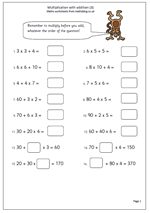# Resource of the Week: year 4 multiplication and additionThis week we are highlighting an important part of year 4 maths which looks at the order of operations.

The rule is that multiplication should be worked out before the addition and in most of these questions that is fairly straightforward. However the questions towards the end are quite tricky.

Let’s look at 70 + ?? x 4 = 150

The best way to go about this is to go in reverse order; subtract 70 from 150 which leaves 80.

Then work out what number multiplied by 4 makes 80, which is 20.

Therefore the missing number is 20.

It is best to check this by going back to the original question; multiply 20 by 4 = 80 and then add 80 and 70 = 150, making the statement true.

Many children will find these very difficult to work out as they require several steps.